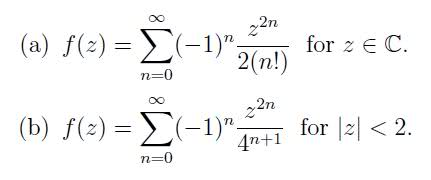# Is there a differnt way to write this expression?

Firepanda
f(z) = SUM [(-1)n

Where SUM = sum from n=0 to infinity

Looks to me like there isn't but there must be a simpler way to write that.

Thanks

Last edited:

Homework Helper
It's divergent, isn't? The nth term doesn't approach 0.

Firepanda
It's divergent, isn't? The nth term doesn't approach 0.

The question is to write this power series as a functionLast edited:
Homework Helper
z^(2n)/n! isn't equal to e^(z^2). The SUM of the series z^(2n)/n! is e^(z^2). That would be fine if the (-1)^n weren't there. Can't you think of a function that's a lot like e^(z^2) that will give you the alternating sign in the series?

Firepanda
I just keep getting into the routine of trying to simply it like before. Do you mean replace e^(z^2) or multiply in some function f(z)/f(z)?

Homework Helper
I just keep getting into the routine of trying to simply it like before. Do you mean replace e^(z^2) or multiply in some function f(z)/f(z)?

(-1)^n*z^(2n). Can you think of a way to write that as r^n for some number r? What would r be?

Firepanda
ahh yes

Last edited:
Firepanda
For the 2nd part I have it down to

z-2 SUM (-1)n.(z2/4)n+1

Is there anyway I can progress from here or should I go back and try a different way?

Homework Helper
For the 2nd part I have it down to

z-2 SUM (-1)n.(z2/4)n+1

Is there anyway I can progress from here or should I go back and try a different way?

It's a geometric series.

Firepanda
It's a geometric series.

(z2/4)n+1 starting at n=0

= 1/(1-z2/4) - 1

=> Series = z-2 SUM (-1)n/(1-z2/4) + (-1)n+1

Homework Helper
(z2/4)n+1 starting at n=0

= 1/(1-z2/4) - 1

=> Series = z-2 SUM (-1)n/(1-z2/4) + (-1)n+1

You just did the same wrong thing you did in the first problem. You replaced a term is the series with the sum of a series. And you missed the cue to do the same thing you did with the (-1)^n in the first problem. You series has the form a*r^n. r just might have a (-1) in it.

Firepanda
ah yes, i have my r now thanks, was simple after that!

Thanks alot